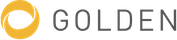# Quantum field theory

Theoretical framework for constructing quantum mechanical models of systems classically represented by an infinite number of degrees of freedom

### All edits

Edits on 7 Feb, 2022
Article (+6524 characters)
Article

In theoretical physics, quantum field theory (QFT) is a theoretical framework that combines classical field theory, special relativity and quantum mechanics.  QFT is used in particle physics to construct physical models of subatomic particles and in condensed matter physics to construct models of quasiparticles.

QFT treats particles as excited states (also called quanta) of their underlying quantum fields, which are more fundamental than the particles. Interactions between particles are described by interaction terms in the Lagrangian involving their corresponding quantum fields. Each interaction can be visually represented by Feynman diagrams according to perturbation theory in quantum mechanics.

History

Quantum field theory emerged from the work of generations of theoretical physicists spanning much of the 20th century. Its development began in the 1920s with the description of interactions between light and electrons, culminating in the first quantum field theory—quantum electrodynamics. A major theoretical obstacle soon followed with the appearance and persistence of various infinities in perturbative calculations, a problem only resolved in the 1950s with the invention of the renormalization procedure. A second major barrier came with QFT's apparent inability to describe the weak and strong interactions, to the point where some theorists called for the abandonment of the field theoretic approach. The development of gauge theory and the completion of the Standard Model in the 1970s led to a renaissance of quantum field theory.

Theoretical background

Quantum field theory results from the combination of classical field theory, quantum mechanics, and special relativity.  A brief overview of these theoretical precursors follows:

The earliest successful classical field theory is one that emerged from Newton's law of universal gravitation, despite the complete absence of the concept of fields from his 1687 treatise Philosophiæ Naturalis Principia Mathematica. The force of gravity as described by Newton is an "action at a distance"—its effects on faraway objects are instantaneous, no matter the distance. In an exchange of letters with Richard Bentley, however, Newton stated that "it is inconceivable that inanimate brute matter should, without the mediation of something else which is not material, operate upon and affect other matter without mutual contact.": 4  It was not until the 18th century that mathematical physicists discovered a convenient description of gravity based on fields—a numerical quantity (a vector) assigned to every point in space indicating the action of gravity on any particle at that point. However, this was considered merely a mathematical trick.

Fields began to take on an existence of their own with the development of electromagnetism in the 19th century. Michael Faraday coined the English term "field" in 1845. He introduced fields as properties of space (even when it is devoid of matter) having physical effects. He argued against "action at a distance", and proposed that interactions between objects occur via space-filling "lines of force". This description of fields remains to this day.

The theory of classical electromagnetism was completed in 1864 with Maxwell's equations, which described the relationship between the electric field, the magnetic field, electric current, and electric charge. Maxwell's equations implied the existence of electromagnetic waves, a phenomenon whereby electric and magnetic fields propagate from one spatial point to another at a finite speed, which turns out to be the speed of light. Action-at-a-distance was thus conclusively refuted.

Despite the enormous success of classical electromagnetism, it was unable to account for the discrete lines in atomic spectra, nor for the distribution of blackbody radiation in different wavelengths. Max Planck's study of blackbody radiation marked the beginning of quantum mechanics. He treated atoms, which absorb and emit electromagnetic radiation, as tiny oscillators with the crucial property that their energies can only take on a series of discrete, rather than continuous, values. These are known as quantum harmonic oscillators. This process of restricting energies to discrete values is called quantization. Ch.2  Building on this idea, Albert Einstein proposed in 1905 an explanation for the photoelectric effect, that light is composed of individual packets of energy called photons (the quanta of light). This implied that the electromagnetic radiation, while being waves in the classical electromagnetic field, also exists in the form of particles.

In 1913, Niels Bohr introduced the Bohr model of atomic structure, wherein electrons within atoms can only take on a series of discrete, rather than continuous, energies. This is another example of quantization. The Bohr model successfully explained the discrete nature of atomic spectral lines. In 1924, Louis de Broglie proposed the hypothesis of wave–particle duality, that microscopic particles exhibit both wave-like and particle-like properties under different circumstances.Uniting these scattered ideas, a coherent discipline, quantum mechanics, was formulated between 1925 and 1926, with important contributions from Max Planck, Louis de Broglie, Werner Heisenberg, Max Born, Erwin Schrödinger, Paul Dirac, and Wolfgang Pauli.

In the same year as his paper on the photoelectric effect, Einstein published his theory of special relativity, built on Maxwell's electromagnetism. New rules, called Lorentz transformation, were given for the way time and space coordinates of an event change under changes in the observer's velocity, and the distinction between time and space was blurred. It was proposed that all physical laws must be the same for observers at different velocities, i.e. that physical laws be invariant under Lorentz transformations.

...

Two difficulties remained. Observationally, the Schrödinger equation underlying quantum mechanics could explain the stimulated emission of radiation from atoms, where an electron emits a new photon under the action of an external electromagnetic field, but it was unable to explain spontaneous emission, where an electron spontaneously decreases in energy and emits a photon even without the action of an external electromagnetic field. Theoretically, the Schrödinger equation could not describe photons and was inconsistent with the principles of special relativity—it treats time as an ordinary number while promoting spatial coordinates to linear operators.

Edits on 1 Mar, 2020
Infobox (+1 properties)
Infobox
Wikidata entity ID
Q54505
Edits on 1 Jan, 2017